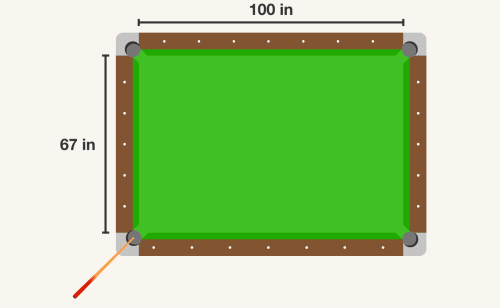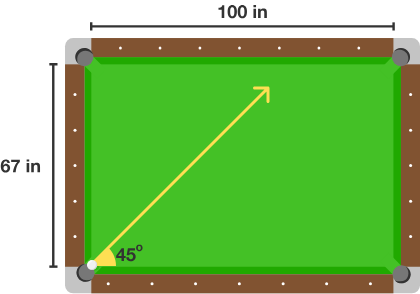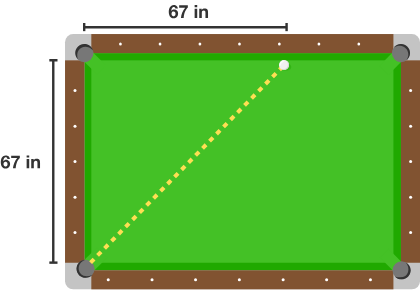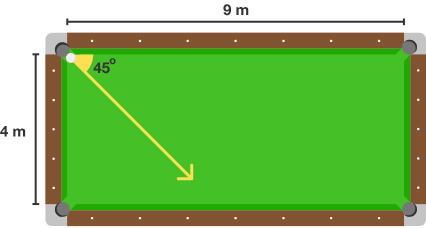## Does It Ricochet Around Forever?

Many people try to line up the shot in yesterday’s challenge using trial and error, guessing and then seeing if it's reasonable when sketched on the figure. That approach doesn't work as well on today's problem.Assume geometric purity: the ball has no mass, the ball and hole are considered single points, and the ball travels straight. What hole will the ball ultimately enter in the animation above (or will it bounce around forever)?

To be precise, we want to consider the situation below: the ball starts in the bottom left corner and is shot at a perfect $$45^\circ$$ angle.To solve this, let's think about the table's dimensions and the characteristics of the 45-degree angle. Because the angle is $$45^\circ,$$ the ball will always move the same distance vertically as it moves horizontally. So the first bounce will occur 67 inches from the left on the top rail.When does it sink in a hole? Only when the ball exactly strikes one of the corners, which means that the horizontal distance needs to be a multiple of 100, and the vertical distance needs to be a multiple of 67 at the same time. Since the vertical and horizontal distances are always equal, the first time this will happen is when the ball has moved 6700 inches both vertically and horizontally. So we know if the ball keeps moving, it will sink in one of the holes… eventually. But which one?

When tracked along the top of the table, the ball moves the full length of the table—100 inches—so after 6700 inches of horizontal movement, it will have traversed the table horizontally 67 times: 34 times going left to right, and 33 times going right to left. Therefore, it will end its movement, falling into a hole, on the right-hand side. We also know that the ball will traverse the table vertically 100 times: 50 times going bottom to top, and 50 times going top to bottom. Therefore, it will end its movement at the bottom of the table. Combining these two constraints, we can conclude that the ball, after a great many bounces, will fall into the bottom right corner pocket.

Can the same reasoning be applied to today's challenge?

# Today's Challenge

Assuming that the ball will travel in a straight line and only enter a hole if it strikes it precisely at the corner, what happens to the ball shown below?×

Problem Loading...

Note Loading...

Set Loading...